Courses

# Central Forces MCQ Level – 1

## 10 Questions MCQ Test Topic wise Tests for IIT JAM Physics | Central Forces MCQ Level – 1

Description
This mock test of Central Forces MCQ Level – 1 for IIT JAM helps you for every IIT JAM entrance exam. This contains 10 Multiple Choice Questions for IIT JAM Central Forces MCQ Level – 1 (mcq) to study with solutions a complete question bank. The solved questions answers in this Central Forces MCQ Level – 1 quiz give you a good mix of easy questions and tough questions. IIT JAM students definitely take this Central Forces MCQ Level – 1 exercise for a better result in the exam. You can find other Central Forces MCQ Level – 1 extra questions, long questions & short questions for IIT JAM on EduRev as well by searching above.
QUESTION: 1

### A spherical shell has mass M and radius R, A point mass m/2 kept inside the shell at a distance R/2 from centre. Then force of attraction on the mass is :

Solution:

Inside spherical shell gravitational field due to shell is zero.

QUESTION: 2

### With what angular velocity the earth should spin in order that a body lying at 37° latitude may become weightless.

Solution:

Due to effect of rotation of earth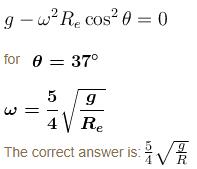QUESTION: 3

### A planet revolving around sun in an elliptical orbit has a constant :

Solution:

In elliptical orbit sun is at one of the foci hence the distance between the planet and sun changes as planet revolves hence linear speed, kinetic energy and potential energy of planet do not remain constant.
Angular momentum about the sun remains constant.

QUESTION: 4

A tunnel is dug along the diameter of the earth (Radius R and mass M). There is particle of mass m  at the centre of the tunnel. Find the minimum velocity given to the particle so that it just reaches to the surface of the earth :

Solution:

Let the minimum speed imparted to the particle of mass  m  so that it just reaches surface of earth is  v.
Applying conservation of energy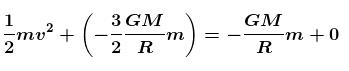Solving we get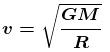The correct answer is: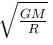QUESTION: 5

A satellite of mass m revolves around the earth of radius R at a height  x from its surface. If is the acceleration due to gravity on the surface of the earth, the orbital speed of the satellite is :

Solution: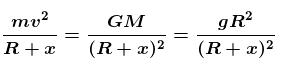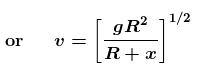The correct answer is: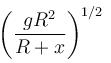QUESTION: 6

Which of the following graph represents the time period of the planet moving around the sun. [R = semi major axis of the path]

Solution:

T2 ∝ R3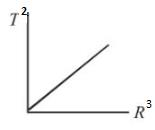QUESTION: 7

At what height above the earth’s surface does the acceleration due to gravity fall to 1% of its value at the earth’s surface?

Solution:

Acceleration due to gravity at a height h from earth surface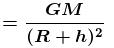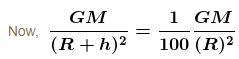⇒ h = 9 R

QUESTION: 8

Two particles, each of mass M move around in a circle of radius R under the action of their mutual gravitational attraction. The speed of each particle is

Solution: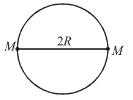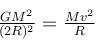⇒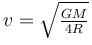The correct answer is: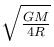QUESTION: 9

A geostationary satellite is one :

Solution:

A geostationary satellite has time period  same as that of earth.

The correct answer is: whose time period is same as that of earth

QUESTION: 10

Three particles each of mass m are kept at vertices of an equilateral triangle of side L. The gravitational field at centre due to these particles is :

Solution:

Let us put a test mass mat the center. Forces acting on it are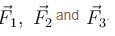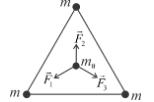These forces are of equal magnitude and act at 120° with each other .
Hence,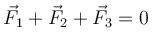∴ Fg = 0